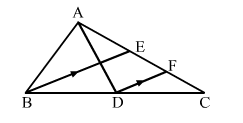# In the adjoining figure, AD and BE are the medians of ∆ABC

Question:

In the adjoining figure, $A D$ and $B E$ are the medians of $\triangle A B C$ and $D F \| B E$. Show that $C F=\frac{1}{4} A C$.Solution:

In  ABC, we have:
AC = AE + EC     ...(i)
AE = EC               ...(ii)       [BE is the median of  ABC]
∴ AC = 2EC          ...(iii)

​In ∆BECDF || BE.
∴ EF = CF        (By midpoint theorem, as D is the midpoint of BC)
But EC = EF CF
⇒ EC =​ 2 ⨯ ​CF       ...(iv)
From (iii) and (iv), we get:
AC = 2 ⨯ (⨯​ CF)

$\therefore C F=\frac{1}{4} A C$